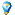###Author Topic: How to find good pairs?  (Read 3705 times)

#### damiano

• Newbie
•• Posts: 8
• Karma: +0/-0##### How to find good pairs?
« on: July 23, 2013, 03:41:54 am »
Hello,
First of all many congratulations for this awesome tool. I have started few months ago with PairTrading but i never found a tool like this. Thank you.

WIth this thread I would like to understand how can I find good pairs to trade.

I did this backtest (https://www.pairtradinglab.com/backtests/Ue2TfX6MxWBuvmrH) their prices seem move togheter but the ratio does not look so good to me, so my questions are:

1. How to find good ratios? (stable, ranging ratios)

2. Looking at the plot I see you started from 2008, it is to much data i think, how many daily periods do you use to calculate the regression coefficients?

3. Which tests do you do to understand if a pair is good to trade? (with good i mean stable/ranging),

4. Maybe is it useful to test for structural breaks in ratio?

« Last Edit: July 23, 2013, 04:00:29 am by damiano »

• Sr. Member
••• Posts: 270
• Karma: +10/-0##### Re: How to find good pairs?
« Reply #1 on: July 23, 2013, 09:32:13 am »
I have inspected your pair GLD-GOLD. I agree with you that prices seem to move together quite nicely, also the correlation is fine, but the pair is not "robust enough" and does not work fine for all model parameters especially in the recent period. The biggest flaw of this pair is the instability and ugly equity curve for most settings
.
This is the whole study for your pair:

So you can see, it is not profitable for all model settings (some points are below zero, at least for the ratio model).

Apparently, residual model works better for this pair, there are even settings exhibiting nice profit:

But I would probably not really trade this pair for reasons stated above. Apparently the contegration is diminishing from time to time. This pair could be better to trade in triplet (3 stocks), but my website currently does not provide tools to test triplets.

Quote
1. How to find good ratios? (stable, ranging ratios)
I suggest not to look to ratios in the first place. Look for the good pairs (not all pair trading models work with ratio). My biggest criteria is sufficient profit on sufficient period of historical data across the whole model settings space + nice linear equity curve + significant positive correlation.

Check this pair for instance:

Check this tutorial too (not complete, but may be useful already):

Quote
2. Looking at the plot I see you started from 2008, it is to much data i think, how many daily periods do you use to calculate the regression coefficients?

Ratio model you used does not work with regression. Ratio is simply calculated as Price1/Price2. Also, you can choose your own time period for the backtest.
In Residual model, linear regression is used (floating window), you can choose the linear regression period.

Quote
3. Which tests do you do to understand if a pair is good to trade? (with good i mean stable/ranging)

Quote
4. Maybe is it useful to test for structural breaks in ratio?

Not sure what do you mean, can you explain?

#### damiano

• Newbie
•• Posts: 8
• Karma: +0/-0##### Re: How to find good pairs?
« Reply #2 on: July 23, 2013, 02:03:23 pm »
I still have two questions:

2. Why are you calculating the ratio using STOCK1 / STOCK2 instead of their returns ?

Thank you!
« Last Edit: July 23, 2013, 02:09:47 pm by damiano »

• Sr. Member
••• Posts: 270
• Karma: +10/-0##### Re: How to find good pairs?
« Reply #3 on: July 25, 2013, 05:50:35 am »
1. Z-Score is always calculated as spread/standard deviation.....if Z-Score is 0, the pair is currently in its mean with no profit potential, if Z-Score is 2 it means the pair is currently two standard deviations from the "mean"

- in Ratio model, spread is calculated as R-MA (R is P1/P2, MA is the moving average of ratios)...standard deviation is calculated from ratio series
- in Residual model, spread is equal to the residuals of the linear regression between P1 and P2 series

2. No particular reasonThis is the simplest method, there are also different approaches (like log(P1)-log(P2))...using P1/P2 is the most used concept used by other pair traders (together with P1-P2, which we don't use)